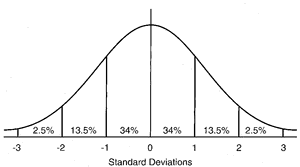# normal distribution

(redirected from Normally distributed)
Also found in: Dictionary, Thesaurus, Financial, Encyclopedia.

## distribution

[dis″trĭ-bu´shun]
1. the specific location or arrangement of continuing or successive objects or events in space or time.
2. the extent of a ramifying structure such as an artery or nerve and its branches.
3. the geographical range of an organism or disease.
frequency distribution in statistics, a mathematical function that describes the distribution of measurements on a scale for a specific population.
normal distribution a symmetrical distribution of scores with the majority concentrated around the mean; for example, that representing a large number of independent random events. It is in the shape of a bell-shaped curve. Called also gaussian distribution. See illustration.Normal distribution. The approximate percentage of the area (or frequency) lying under the curve between standard deviations is indicated. From Dorland's, 2000.
probability distribution a mathematical function that assigns to each measurable event in a sample group the probability that the event will occur.

## nor·mal dis·tri·bu·tion

a specific bell-shaped frequency distribution commonly assumed by statisticians to represent the infinite population of measurements from which a sample has been drawn; characterized by two parameters, the mean (x) and the standard deviation (σ), in the equation:

## normal distribution

A bell-shaped frequency distribution of data, the plotted curve of which is symmetrical about the mean, indicating no significant deviation of the data set from the mean.

Properties of a normal distribution
Continuous and symmetrical, with both tails extending to infinity; arithmetic mean, mode, and median are identical. The curve’s shape is completely determined by the mean and standard deviation.

## gaus·si·an dis·tri·bu·tion

(gow'sē-ăn dis'tri-byū'shŭn)
The statistical distribution of members of a population around the population mean. In a gaussian distribution, 68.2% of values fall within ± 1 standard deviation (SD); 95.4% fall within ± 2 SD of the mean; and 99.7% fall within ± 3 SD of the mean.
Synonym(s): bell-shaped curve, normal distribution.

## normal distribution

Gaussian distribution, a distribution which when expressed graphically is bell-shaped. The distribution to which many frequency distributions of biological variables, such as height, weight, intelligence, etc correspond.

## Gauss,

Johann K.F., German physicist, 1777-1855.
gauss - a unit of magnetic field intensity.
gaussian curve - a specific bell-shaped frequency distribution. Synonym(s): gaussian distribution; normal distribution
gaussian distribution - Synonym(s): gaussian curve

## nor·mal dis·tri·bu·tion

(nōr'măl dis'tri-byū'shŭn)
Specific bell-shaped frequency distribution commonly assumed by statisticians to represent the infinite population of measurements from which a sample has been drawn.
References in periodicals archive ?
If the observations are not normally distributed or the sample size is not large enough, non-parametric analyses (e.g., Mann-Whitney U test instead of a t-test and Kruskal-Wallis H test instead of a one-way ANOVA) would be the methods of choice.
Shapiro-Wilk univariate normality tests indicated that BDI scores were not normally distributed either the pretest or the posttest (Table 1).
The remainder of this paper is organised as follows: Section 2 reviews random decision-making and related algorithms in which attribute values are normally distributed stochastic variables.
An unpaired two- tailed i-test based on ranks (Mann-Whitney) was used to compare differences between the two groups for data that were not normally distributed (a) and a two-tailed unpaired student's i-test was used for normally distributed data (b).
For normally distributed measurement errors, 68.3% of the possible observations lie between the values, mean [+ or -] SD.
The normally distributed random errors and outliers in observations also produce data jumps, whose magnitudes are examined by the model of pseudo normally distributed random errors described in the section 3.1 above.
Based on Figure 4, the histogram shows that there is a bell-shaped indicating that the error terms are normally distributed. It can be confirmed by looking at the skewness of the distribution in Table 2.
This means that we cannot assume that the data are normally distributed. Thus, to apply the Student's t-test would be not properly.
He has found that daily and weekly returns are not normally distributed because they are negatively skewed and leptokurtic.
For continuous variables, Student's t-test was used to compare means of normally distributed variables, while non-normally distributed variables were subjected to Mann-Whitney U test for comparison of medians.
The percentage difference between normally distributed variables is not a normally distributed variable itself, however, as it involves a ratio of 2 normally distributed variables, as previously described by Marsaglia (8, 9).

Site: Follow: Share:
Open / Close